# PHP笔记

+关注继续查看

1.php4种标签风格及3种注释风格

i.标签风格

a.XML风格

<?php

echo "hello world!";

?>

b.ASP风格

<%

echo "hello world!";

%>

c.脚本风格

<script language="php">

echo "hello world!";

</script>

d.极简风格

<?echo "hello world!";?>

ii.注释风格

a.c++风格的单行注释 //

b.c++风格的多行注释 /**/

c.shell风格的注释 #

2.php脚本执行的3种参数传递与解析

a.$argc和$argv 传递参数

<?php

echo "接收到{$argc}个参数"; print_r($argv);

?>

paras count : 5Array

(

 => .\hello.php

 => hello

 => world

 => -a

 => 123

)

b.getopt()函数解析参数

<?php

$param_arr = getopt('a:b:c:'); print_r($param_arr);

?>

Array

(

[a] => 345

[b] => 12q3

)

c.提示用户输入

<?php

fwrite(STDOUT,'请输入您的博客名：');

echo '您输入的信息是：'.fgets(STDIN);

?>

3.php数据类型

php的变量使用$开头，属于动态数据类型，数据类型包括： boolean string integer float array object resource null值 数据类型的定义： a.string 单引号和双引号字符串的区别 同shell中的单双引号的区别相同，双引号的字符串需要先执行递归的变量替代$a='hello world';

echo "hi,$a"; b1.string的界定符输出 目的：增强可读性$string=<<<std

hi,

hello world

std; //结束

b2.格式化字符串

$formatted = sprintf ("hello,%s", "world"); printf($formatted);

c.array3种定义

$array=array("v1","v2");//数值自动键，从0开始$array[key]="vkey";//字符指定键

$array=array(key1=>v1,key2=>v2) //字符键和数字键可以混用 d.null值 通常会用于变量申明$str=null;

is_null($str) //true$str="str"

is_null($str) //false unset($str)

is_null($str) //true e.数据类型之间的强制转换$str="123"

$num=(integer)$str

$bl=(boolean)$str

f.数据类型检测函数

is_bool(),is_string,is_float(),is_double(),is_integer(),is_int(),is_null(),is_array(),is_object(),is_numeric()

g.常量定义函数

define(string 常量名,值,true/false大小写敏感)

bool defined(string 常量名) //判断该常量是否已经定义

define("ms",123)

echo ms+24;

h.php预定义常量

__FILE__  PHP程序文件名

__LINE__  PHP当前文件行数

PHP_VERSION

PHP_OS    执行PHP的OS

...

i.string的+运算和。运算

string + ：表示将字符串转换成数字再执行+  (只转换字符串首部的数字，首部为字母则结果为0)

string . ：表示将字符串拼接

$n="3.14r";$m=1;

echo $n.$m , $n+$m  //3.14r1  4.14

4.变量

a.变量的引用定义&

$i="hello"$j=&$i$i="$i,world" echo$i  // hello,world

echo $j // hello,world b.变量的3种作用域 i.局部变量 函数中定义的变量，作用域为整个函数 ii.全局变量 函数以外的变量，作用域为当前PHP文件，但函数内部不可用，除非在函数中申明global才可作用于函数中 iii.静态变量 定义于函数中，函数结束时不会被释放 <?php$msg="hello"; //全局变量

function hello(){

static $msg1="hello";//静态变量 global$msg; //函数中使用全局变量

$msg1="hello" //局部变量 } ?> c.变量的递归性（可变变量）$a="hello,world";

$b="a" echo$$b //将输出hello,world d.php预定义变量$_SERVER['SERVER_ADDR'] //当前运行脚本所在的服务器IP

$_SERVER['SERVER_NAME'] //...服务器名$_SERVER['REQUEST_METHOD'] //请求方法

$_SERVER['REMOTE_ADDR'] //$_SERVER['REMOTE_HOST'] //

$_SERVER['REMOTE_PORT'] //$_SERVER['SCRIPT_FILENAME'] //当前脚本的绝对路径

$_SERVER['SERVER_PORT'] //$_SERVER['DOCUMENT_ROOT'] //当前脚本的文档根目录

$_COOKIE //通过HTTPCookie传递到脚本的信息（数组）$_SESSION //包含与所有回话有关的信息（数组）

$_POST //包含通过POST方法传递的相关信息（数组）$_GET //包含通过GET方法传递的相关信息（数组）

$_GLOBALS //所以已定义的全局变量组成的数组，变量名是该数组的索引（数组） 5.运算符 c语言中的所有运算符适用于PHP，并有其他运算符： @：错误屏蔽运算符，可以屏蔽表达式产生的错误$err=@(5/0) ;//防止系统报错，使得程序可以继续运行

6.函数

a.函数定义与调用

function fun_name($arg1,&$arg2,$arg3=value,...){ } function exap($num){

return $num+10; } echo exap(10); 特点：入参的传递有2种值传递和引用传递,参数可以有默认值 b.函数作为变量值时的调用(变量函数)$fun="exap";

$fun(10); c.函数返回值得引用<=>函数的引用$a=&exap(10)

echo $a."<p>"; unset($a) //解引用或解变量

7.流控制语句

a.if,switch,while,do...while,for与C语言相同

b.foreach 用于数组和对象

foreach(array_expression as $value) 或者 foreach(array_expression as$key => $value) 8.字符串处理 a.trim(string str[,string charlist]),ltrim,rtrim 默认删除字符串左右两边所有的空格,\0,\t,\n,\x0B,\r b.字符串转义 "\",addcslashes(),stripcslashes() 例子：$m1="select * from t where book='m'";

$m="select * from t where book=\'m\'";$m=addcslashes($m1) 也可以去转义 stripcslashes($m)

c.子字符串

string substr(string str,int start[,int len])

d.比较字符串

int strcmp(string str1,string str2)

int strnatcmp(string str1,string str2) 不区分大小写

int strncmp(string str1,string str2,int len)

e.字符串查找

string strstr(string str,string findstr) 返回findstr第一次出现的位置到str末尾的子字符串

int substr_count(string str,string findstr) 查找子字符串出现的次数

f.字符串替代

string str_ireplace(string new_substr,string old_substr,string str[,int count替代次数])

string substr_replace(string str,string repl,int star[,int len])

g.分割字符串

array explode(string sep,string str[,int limit限制返回值最多包含的元素])

h.合成字符串

string implode(string glue,array)

9.正则表达式

a.bool ereg/eregi(string pattern,string str[,returnarray]) 在字符串str中匹配pattern模式， 将匹配的字符放在返回数组中；如果匹配成功返回true，否则返回false。

b.string ereg_replace/eregi_replace(string pattern,string rep,string str) 使用rep替代 str中所有匹配pattern的子串，返回最后替代的结果

c.array split/spliti(string pattern,string str[,int limit]) 使用pattern分割str，返回 分割后的数组，最后返回limit个元素

d. array preg_grep(string pattern ,array input) 使用pattern匹配数组array中的所有元素， 返回完全匹配的元素（而不是部分匹配）;pattern必须使用"/.../"包围

e.preg_match/preg_match_all(string pattern,string str [,array matches]) 使用pattern 匹配str中所有的子串并返回，返回匹配的次数

f. string preg_quote(string str[,string delimiter]) 将str中的所有特殊字符自动转义， delimiter字符串指定的所有字符也将被转义(即加上一个‘\’)

g.mixed preg_replace(mixed pattern, mixed replacement,mixed str[,int limit])  使用pattern在str中匹配，将匹配的子串替代为replacement，最多替代limit次

h.mixed preg_replace(mixed pattern, callback ck,mixed str[,int limit])  与上述函数相同，但是是使用ck函数的返回值来替代，ck的入参是一个数组，该数组是每一个匹配返回的所有分组

i.array preg_split(string pattern,string str[,int limit]) 使用pattern模式分割str。

10.数组

print_r(mixed)可以打印数组

each()和list()

explode()字符串拆分成数组

implode()数组组合成字符串

int count(array[,int mode]) 统计数组元素个数（mode==1递归统计）

mixed array_search(key,array) 在array中查找键值，如果找到返回键值；否则返回FALSE

mixed array_pop(array) 获取并删除数组中的最后一个元素，如果数组为空则返回null

int array_push(array,mixed v1[,v2,...]) 向数组尾部插入元素，并返回数组中的元素总数

array array_unique(array) 删除数组中重复的元素

11.php与web表单交互数据

a.表单用于浏览器发送数据到服务器，并使用action指明使用哪个url进行表单数据的处理。浏览器的数据只能通过表单来传送。表单的元素较多：checkbox,submit,reset,button,textarea...

b.GET和POST提交表单的区别：使用GET提交的表单将会将表单数据作为参数附加到url中进行提交；而POST方法不会。

c.关于全局变量$_POST,$_GET,$_SESSION$_POST:数组格式，POST方法传递表单，使用表单元素的元素名获取元素值：$_POST['user'] .$_GET :数组格式，GET方法传递表单，使用表单元素的元素名获取元素值：$_GET['user']$_SESSION:session传参获取变量。使用表单元素的元素名获取元素值：$_SESSION['user'] 根据表单元素的不同，传回来的'user'键对应的值有可能是一个数组 d.在web中嵌入php脚本的方法只有2种： 使用HTML标记<?php...?>编写php脚本;对表单元素的value属性进行赋值 <?php include("index.php");//可以引用外部文件解耦合,多次引用将多次包含，如果只引用一次可以使用require_once require_once("index.php"); //require ?> <?php$hidden="yg0025";

?>

<input type="hidden" name="ID" value="<?php echo $hidden;?>">$re=require("...")函数及其变种都具有返回值，返回调用的php文件中return函数的值

<?php

return "hello";

?>

include 和require的执行过程都是先将代码原本的拷贝到当前文件再执行。

return的效果也可以理解成将代码返回。return后面的代码将不会被拷贝。

12.php与JavaScript交互

a.js的一些常识

js是一种由浏览器运行解析的脚本（也可以由服务器执行）。可以在不与服务器交互的条件下实现动态网页。

js事件相应模式：<input type="submit" name="submit" value="检测" onClick="check()">

js的常用事件：onclick,ondblclick,onmousedown,...可以在网上查询js常用事件

13.日期与时间

<?php

?>

session

<?php

session_start();

$_SESSION["admin"]="hello";//完成写session ?> 读session <?php if(!empty($_SESSION['admin'])) //将判断cookie中是否存在session_id和‘admin’字段，否则均返回null

$user=$_SESSION['admin']

?>

unset($_SESSION['admin']);$_SESSION=array();//删除所有session

session_destory();//彻底销毁会话，确定会话结束时，应该这么做

<?php

session_start();

$_SESSION["admin"]='hello' ?> ii.setcookie设置session_id的过期时间 <?php session_start(); setcookie(session_name(),session_id(),time()+60,'/');$_SESSION["admin"]='hello'

?>

c.session只能使用当前session，如果想用上一个session，那是不可能的，因为无法得知session id。

d.session_id的4种传递方式

ii.设置php.ini中的session.use_trans_sid=1,让PHP自动跨页面传递session_id

iii.通过GET方法，隐藏表单传递session_id

iv.使用文件或者数据库存储session_id，在页面间传递中手动调用

15.php的图形图像处理技术

16.php文件处理

17.php面向对象

a.定义

final class demo{

public function __construct(){          //构造函数

echo __METHOD__.":".__LINE__."\n";

}

public function func($param){ //普通函数$param++;

echo "method func $paramn"; } public function __destruct(){ //析构函数 echo __METHOD__.":".__LINE__."\n"; echo$this->sex;

}

//public $name = 123+456; 错误：初始化时不能带有计算 static$a = '$a'; const A = 'A'; public$name = '凤姐';

protected $sex = '男+女'; private$age = 23;

}

{

require_once $class_name.'.php'; } //当前页面 Pserson 类不存在则自动调用 __autoload() 方法，传入参数 Person$p1 = new Person("张三","20");

$p1 -> say(); ?> b3.__get() 当调用一个未定义的属性时，此方法会被触发，传递的参数是被访问的属性名。 __set() 给一个未定义的属性赋值时，此方法会被触发，传递的参数是被设置的属性名和值。 这里的没有声明包括当使用对象调用时，访问控制为proteced,private的属性(即没有权限访问的属性)。 __isset() 当在一个未定义的属性上调用isset()函数时调用此方法 __unset() 当在一个未定义的属性上调用unset()函数时调用此方法 <?php class Person { private$name;

private $sex; private$age;

//__set()方法用来设置私有属性

function __set($property_name,$value) { //在属性赋值时会被调用

echo "在直接设置私有属性值的时候，自动调用了这个 __set() 方法为私有属性赋值<br />";

$this->$property_name = $value; } //__get()方法用来获取私有属性 function __get($property_name) {

echo "在直接获取私有属性值的时候，自动调用了这个 __get() 方法<br />";

return isset($this->$property_name) ? $this->$property_name : null;

}

}

$p1=new Person();$p1->name = "张三";

echo "我的名字叫：".$p1->name; ?> 运行该例子，输出： 在直接设置私有属性值的时候，自动调用了这个 __set() 方法为私有属性赋值 在直接获取私有属性值的时候，自动调用了这个 __get() 方法 我的名字叫：张三 b4.__call($method, $arg_array ) 当调用一个未定义的方法是调用此方法,这里的未定义的方法包括没有权限访问的方法;如果方法不存在就去父类中找这个方法，如果父类中也不存在就去调用本类的__call()方法，如果本类中不存在__call()方法就去找父类中的__call()方法。 b5.__clone()如果想复制一个对象则需要使用clone方法 如果想在克隆后改变原对象的内容，需要在类中添加一个特殊的 __clone() 方法来重写原本的属性和方法。__clone() 方法只会在对象被克隆clone的时候自动调用。 <?php class Person { private$name;

private $age; function __construct($name, $age) {$this->name = $name;$this->age = $age; } function say() { echo "我的名字叫：".$this->name;

echo " 我的年龄是：".$this->age."<br />"; } function __clone() {$this->name = "我是假的".$this->name;$this->age = 30;

}

}

$p1 = new Person("张三", 20);$p1->say();

$p2 = clone$p1;

$p2->say(); ?> b6. __toString()方法在将一个对象转化成字符串时自动调用，比如使用echo打印对象时 __sleep() 串行化的时候用 __wakeup 反串行化的时候调用 __set_state()当调用var_export()时，这个静态 方法会被调用(自PHP 5.1.0起有效) __invoke当尝试以调用函数的方式调用一个对象时，__invoke 方法会被自动调用。 __callStatic(PHP 5.3.0以上版本有效) 是为了处理静态方法调用 c.继承与多态 c1.范围解析操作符（::） 作用：范围解析操作符或者更简单地说是一对冒号，可以用于访问静态成员，类常量，还可以用于覆盖类中的属性和方法。self，parent 和 static这三个特殊的关键字是用于在类定义的内部对其属性或 方法进行访问的。范围解析操作符（::）与类名连用，可以用于访问静态成员、方法和常量；与parent连用，可以访问被覆盖类中的成员和方法。 <?php class MyClass { protected function myFunc() { echo "MyClass::myFunc()\n"; } } class OtherClass extends MyClass { // 覆盖了父类的定义 public function myFunc() { // 但还是可以调用父类中被覆盖的方法 parent::myFunc(); echo "OtherClass::myFunc()\n"; } }$class = new OtherClass();

$class->myFunc(); /** * 输出结果 -> * MyClass::myFunc() * OtherClass::myFunc() */ function mycall(MyClass$a){  //函数的入参可以指明类型也可以不指明

$a->myFunc(); } mycall(new OtherClass()); 多态 ?> c2.接口interface与实现implements class 子类 extends 父类 implemtns 接口1, 接口2, ... { ...... } interface User{ function getDiscount(); function getUserType(); } //VIP用户 接口实现 class VipUser implements User{ // VIP 用户折扣系数 private$discount = 0.8;

function getDiscount() {

return $this->discount; } function getUserType() { return "VIP用户"; } } d.对象的序列化与反序列化->存储 序列化完整过程包括两个步骤：一个是序列化，就是把对象转化为二进制的字符串，serialize() 函数用于序列化一个对象；另一个是反序列化，就是把对象被序列转化的二进制字符串再转化为对象，unserialize() 函数来反序列化一个被序列化的对象。这样整个过程下来，对象内的类型结构及数据都是 完整的。 <?php$p1 = new Person("张三", 20);

$p1_string = serialize($p1);

//将对象序列化后写入文件

$fh = fopen("p1.text", "w"); fwrite($fh, $p1_string); fclose($fh);

$p2 = unserialize(file_get_contents("p1.text"));$p2 -> say();

?>

18.php加密技术

19.php操作mysql数据库

mysql_select_db(string dbname[,resource conn]);//选择使用的数据库 mysql_query("dbname"[,conn])

$result=mysql_query(string query[,resource conn]);//适合所有sql语句，语句中不能加";"$info=mysql_fetch_array($result[,int result_type]); 从数组结果中获取递归一行信息，返回数组;如果是select则$info的键为字段的名字，如果到底尾部则false

$info=mysql_fetch_object($result[,int result_type]); 获取一行作为对象，成员为字段名

$info=mysql_fetch_row($result[,int result_type]); 返回数组，但是是以下标索引的。

int mysql_num_rows()获取查询结果中总的记录数

20.php的PDO

a.支持的数据库

PDO支持MS SQL SERVER,MYSQL,ODBC,ORACLE,POSTGRE SQL等。

b.当数据库发生变化时只需要修改PDO的DSN数据源名称

c.PDO连接mysql

$dbh = new PDO('mysql:host=localhost;dbname=test', 'root', 'nsfocus123');$dbh->setAttribute(PDO::ATTR_ERRMODE, PDO::ERRMODE_EXCEPTION);

$sql = "INSERT INTO user VALUES ('5', 'xiaohua');";$stmt = $dbh->prepare($sql);

$stmt->execute(); echo$dbh->lastinsertid();

$sql = "SELECT * FROM user";$stmt = $dbh->prepare($sql);

$stmt->execute(); while($row = $stmt->fetch(PDO::FETCH_ASSOC)){ // print_r($stmt->fetchAll()  以列名为索引的数组

print_r($row); } 关于fetch的参数取值： PDO::FETCH_ASSOC 从结果集中获取以列名为索引的关联数组。 PDO::FETCH_NUM 从结果集中获取一个以列在行中的数值偏移量为索引的值数组。 PDO::FETCH_BOTH 这是默认值，包含上面两种数组。 PDO::FETCH_OBJ 从结果集当前行的记录中获取其属性对应各个列名的一个对象。 PDO::FETCH_BOUND 使fetch()返回TRUE，并将获取的列赋给在bindParm()方法中指 定的相应变量。 PDO::FETCH_LAZY 创建关联数组和索引数组，以及包含列属性的一个对象，从而可以在这三种接口中任选一种。 21.ThinkPHP架构 22.一些有用的函数 a.trigger_error("输出信息", E_USER_ERROR);触发fatal错误，并退出程序执行 b.exit(0); 退出当前进程 c.$pid = pcntl_fork(); 创建子进程，需要pcntl模块

23.php的模块安装方法

a.下载需要安装的源码，编译生成.so或.dll

b.将动态库复制到php安装目录下的ext目录中

c.修改安装目录下的php.ini配置文件，在[php]字段中添加 extension=***.so

e.php -m |grep *** 查看模块是否安装成功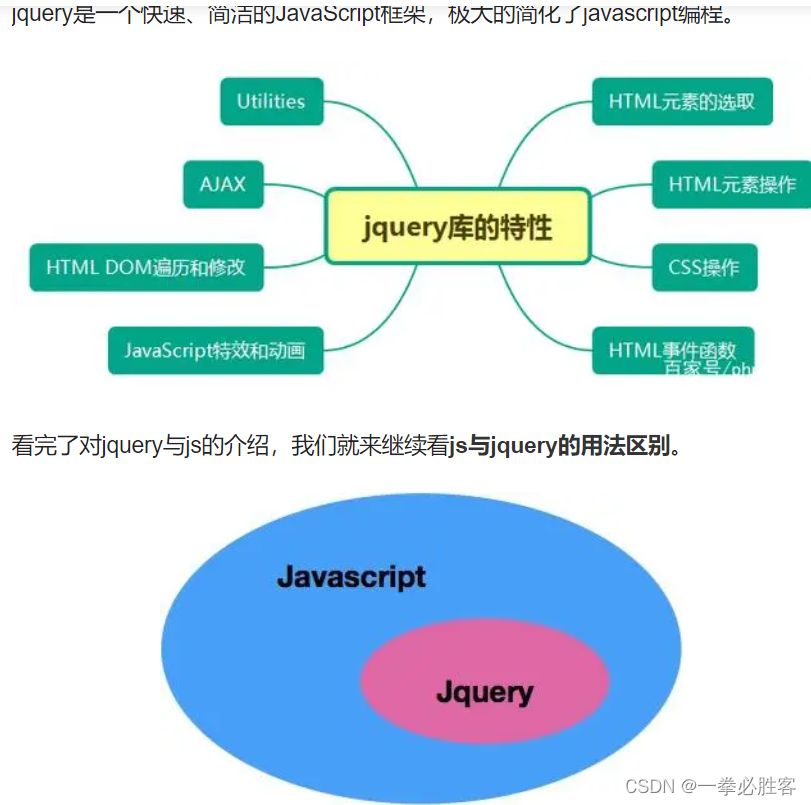【php详细笔记】上传文件到服务器（下）
【php详细笔记】上传文件到服务器
57 0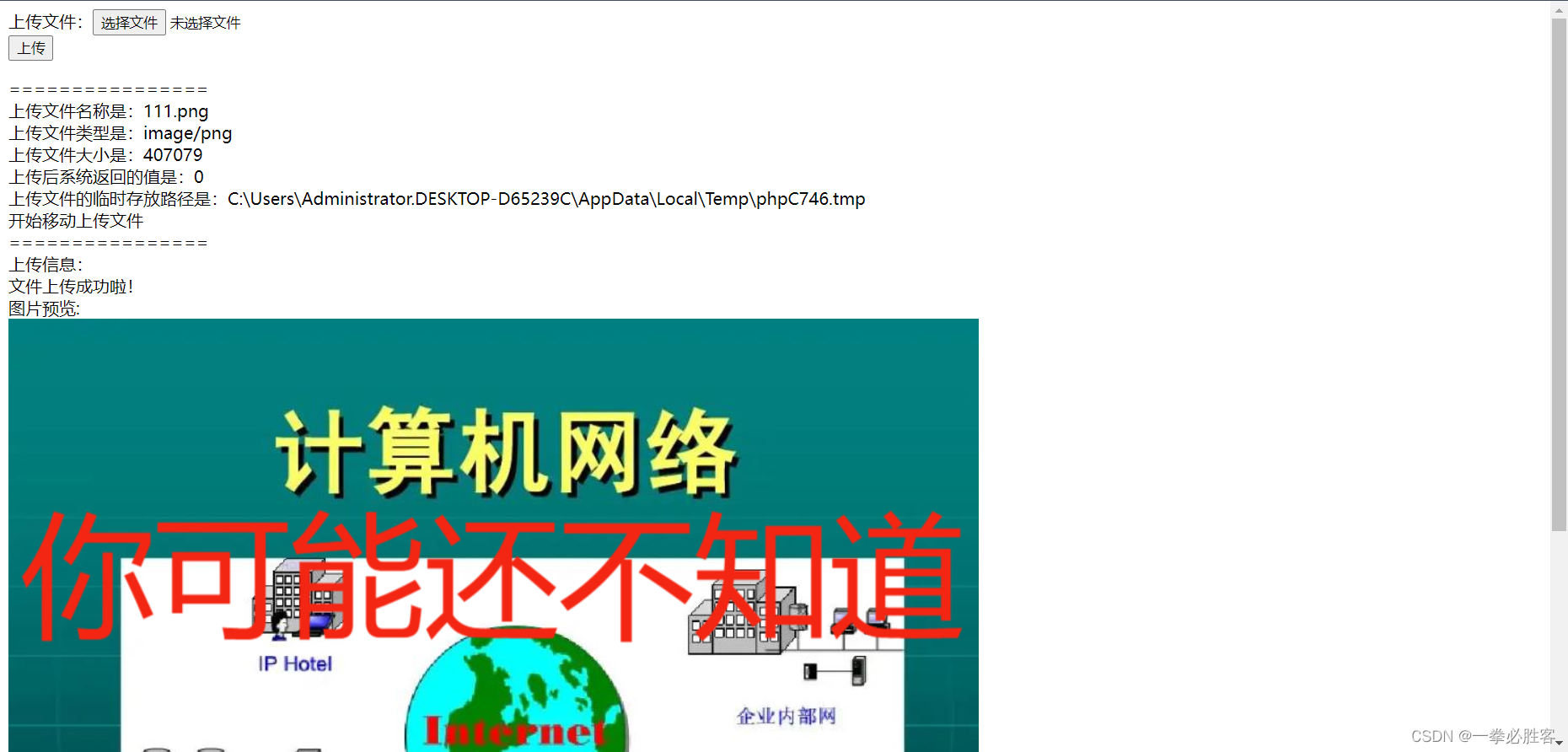【php详细笔记】上传文件到服务器（上）
【php详细笔记】上传文件到服务器
86 0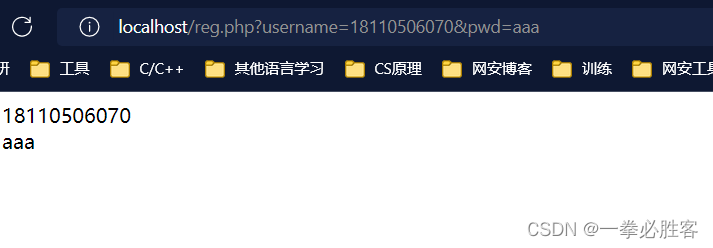【PHP快速入门】详细笔记---精简版（下）
【PHP快速入门】详细笔记---精简版
54 0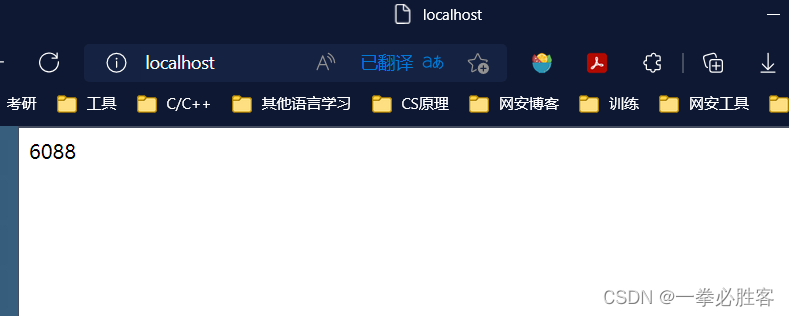【PHP快速入门】详细笔记---精简版（上）
【PHP快速入门】详细笔记---精简版
58 0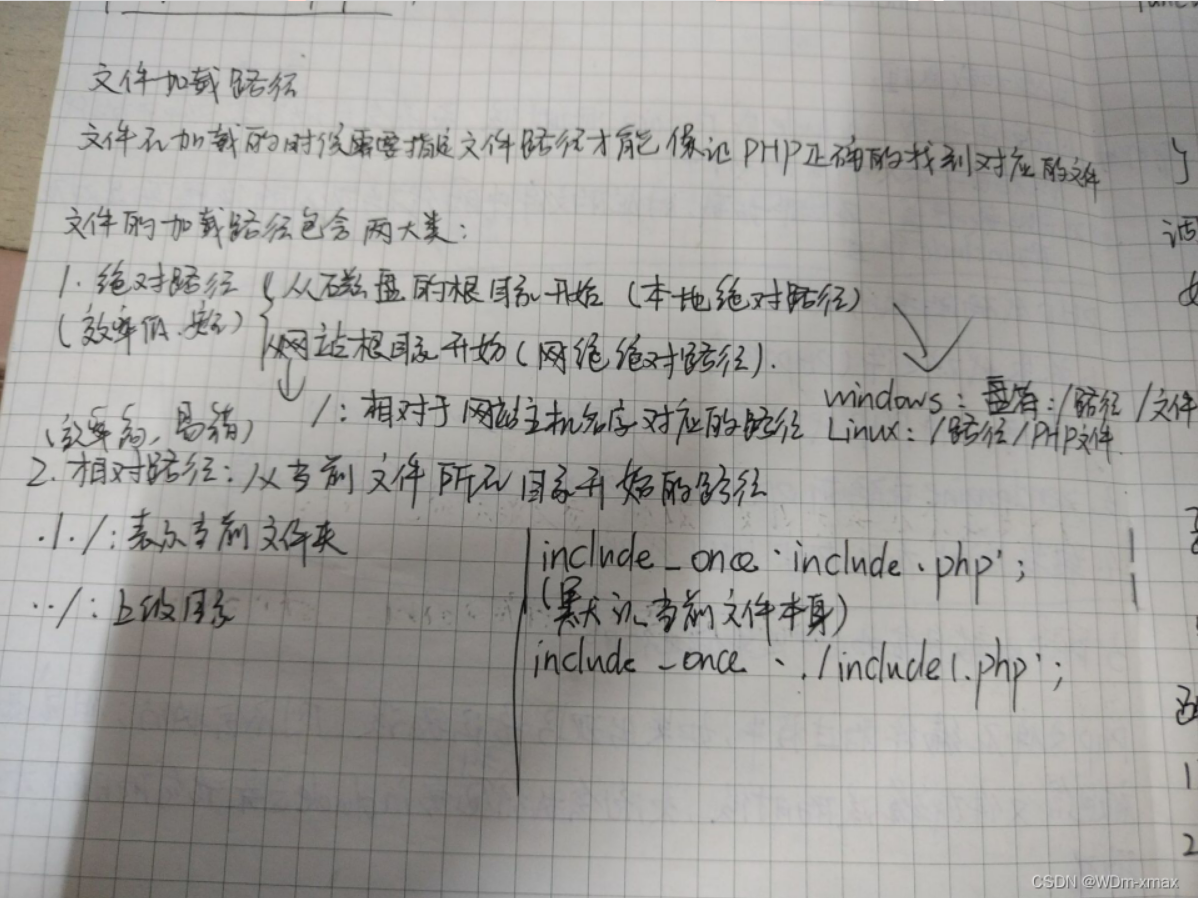PHP基础笔记-NO.4
PHP基础笔记
30 0PHP基础笔记-NO.3
PHP基础笔记
43 0PHP基础笔记-NO.2
PHP基础笔记
35 0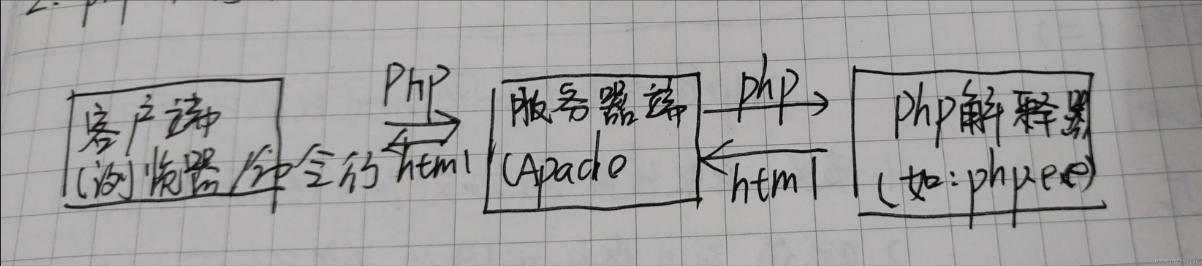PHP基础笔记-NO.1
PHP基础笔记
43 0PHP 零基础入门笔记（15）：算法 algorithm
PHP 零基础入门笔记（15）：算法 algorithm
49 0

PHP完全自学手册文档教程946188

PHP 2017.北京 全球开发者大会——高可用的PHP